# Aligned Induction

## Induced modelling of digit

MNIST - handwritten digits/Induced modelling of digit

### Sections

Introduction

15-fud model

All pixels

Averaged pixels

Array of square regions of 15x15 pixels

Two level over 10x10 regions

Two level over 15x15 regions

Two level over centred square regions of 11x11 pixels

### Introduction

We have considered using just the substrate variables to predict digit, $V_{\mathrm{k}} \to V_{\mathrm{l}}$, by minimising the label entropy or query conditional entropy. See Entropy and alignment. We found that, overall, of all of these models the 511-mono-variate-fud has the highest accuracy of 75.0%.

We have gone on to consider various ways of creating an unsupervised induced model $D$ on the query variables, $V_{\mathrm{k}}$, which exclude digit. Now we shall analyse this model, $D$, to find a semi-supervised submodel that predicts the label variables, $V_{\mathrm{l}}$, or digit. That is, we shall search in the decomposition fud for a submodel that optimises conditional entropy.

There are some examples of model induction in the NIST repository.

### 15-fud model

We have created a 15-fud induced model, $D$, over all pixels NIST_model2.json of 7,500 events of the training sample, see Model 2 and Model 2.

:l NISTDev

(uu,hrtr) <- nistTrainBucketedIO 2

let digit = VarStr "digit"
let vv = uvars uu
let vvl = sgl digit
let vvk = vv minus vvl

let hr = hrev [i | i <- [0.. hrsize hrtr - 1], i mod 8 == 0] hrtr

df <- dfIO "./NIST_model2.json"

let uu1 = uu uunion (fsys (dfff df))

card $dfund df 147 card$ fvars $dfff df 542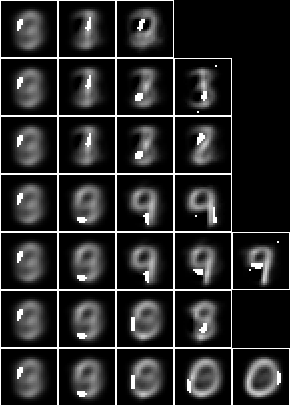This induced model is not interested particularly in digit, but the leaf nodes tend to be resemble particular digits nonetheless. Consider this model as a predictor of label. Create the fud decomposition fud,$F = D^{\mathrm{F}}$, let ff = dfnul uu1 df 1 let uu2 = uu uunion (fsys ff) let hrb = hrfmul uu2 ff hr  The label entropy,$\mathrm{lent}(A * \mathrm{his}(F^{\mathrm{T}}),W_F,V_{\mathrm{l}})$, where$W_F = \mathrm{der}(F)$, is hrlent uu2 hrb (fder ff) vvl 1.3524518741683345  Load the test sample and select a subset of 1000 events$A_{\mathrm{te}}$, (_,hrte) <- nistTestBucketedIO 2 let hrq = hrev [i | i <- [0 .. hrsize hrte - 1], i mod 10 == 0] hrte let hrqb = hrfmul uu2 ff hrq  The conditional entropy decomposition fud of a bi-valent substrate is mostly effective,$\mathrm{size}((A_{\mathrm{te}} * F^{\mathrm{T}}) * (A * F^{\mathrm{T}})^{\mathrm{F}}) \approx \mathrm{size}(A_{\mathrm{te}})$, size$ hhaa (hrhh uu2 (hrqb hrhrred (fder ff))) mul eff (hhaa (hrhh uu2 (hrb hrhrred (fder ff))))
986 % 1


Overall, this model is correct for 46.3% of the test sample, $\begin{eqnarray} &&|\{R : (S,\cdot) \in A_{\mathrm{te}} * \mathrm{his}(F^{\mathrm{T}})~\%~(W_F \cup V_{\mathrm{l}}),~Q = \{S\}^{\mathrm{U}}, \\ &&\hspace{4em}R = A * \mathrm{his}(F^{\mathrm{T}})~\%~(W_F \cup V_{\mathrm{l}}) * (Q\%W_F),~\mathrm{size}(\mathrm{max}(R) * (Q\%V_{\mathrm{l}})) > 0\}| \end{eqnarray}$

let amax = llaa . take 1 . reverse . map (\(a,b) -> (b,a)) . sort . map (\(a,b) -> (b,a)) . aall . norm . trim

length [rr | let hhq = hrhh uu2 (hrqb hrhrred (fder ff union vvl)), let aa = hhaa (hrhh uu2 (hrb hrhrred (fder ff union vvl))), (_,ss) <- hhll hhq, let qq = single ss 1, let rr = aa mul (qq red fder ff) red vvl, size rr > 0, size (amax rr mul (qq red vvl)) > 0]
463


So the accuracy is higher than the 5-tuple (44.8%) but considerably lower than the 1-tuple 15-fud (56.7%).

We can test the accuracy of model 2 in a compiled executable, see NIST test, with these results,

model: NIST_model2
selected train size: 7500
model cardinality: 542
nullable fud cardinality: 711
nullable fud derived cardinality: 143
nullable fud underlying cardinality: 147
ff label ent: 1.3524518741683345
test size: 1000
effective size: 986 % 1
matches: 463


The underlying of the 15-mono-variate-fud conditional entropy model is just the query substrate, $V_{\mathrm{k}}$. Now let us consider taking the fud decomposition fud variables, $\mathrm{vars}(F)$, of the induced model, $F = D^{\mathrm{F}}$, as the underlying. First we must reframe the fud variables $F_1$,

let refr1 (VarPair (VarPair (f, l), i)) = VarPair (VarPair (VarPair (VarInt 1, f), l), i)
refr1 v = v

let tframe f tt = fromJust $transformsMapVarsFrame tt (Map.fromList$ map (\v -> (v, f v)) $Set.toList$ tvars tt)
fframe f ff = qqff (Set.map (\tt -> tframe f tt) (ffqq ff))

let ff1 = fframe refr1 ff

let uu1 = uu uunion (fsys ff1)



Now we apply the reframed fud to the sample, $A_1 = A * \mathrm{his}(F_1^{\mathrm{T}})$,

let hr1 = hrfmul uu1 ff1 hr



Now apply the conditional entropy fud decomper to minimise the label entropy. We will construct a 15-fud conditional model over the 15-fud induced model, $\{D_2\} = \mathrm{leaves}(\mathrm{tree}(Z_{P,A_1,\mathrm{L,D,F}}))$,

let decompercondrr vvl uu aa kmax omax fmax = fromJust $parametersSystemsHistoryRepasDecomperConditionalFmaxRepa kmax omax fmax uu vvl aa let (kmax,omax) = (1, 5) let (uu2,df2) = decompercondrr vvl uu1 hr1 kmax omax 15 rp$ dfund df2
"{<8,15>,<24,14>,<<<1,2>,n>,2>,<<<1,4>,n>,3>,<<<1,4>,1>,21>,<<<1,5>,1>,6>,<<<1,6>,1>,29>,<<<1,11>,1>,19>,<<<1,11>,2>,14>,<<<1,11>,2>,48>,<<<1,12>,1>,41>,<<<1,13>,1>,5>,<<<1,13>,1>,27>,<<<1,14>,1>,25>,<<<1,15>,n>,9>}"

card $dfund df2 15 let dfll = qqll . treesPaths . dfzz rpln$ map (map (\(_,ff) -> fund ff)) $dfll df2 "[{<<<1,2>,n>,2>},{<<<1,4>,n>,3>},{<<<1,11>,2>,48>}]" "[{<<<1,2>,n>,2>},{<<<1,4>,n>,3>},{<<<1,14>,1>,25>}]" "[{<<<1,2>,n>,2>},{<<<1,4>,n>,3>},{<<<1,15>,n>,9>},{<<<1,11>,2>,14>},{<<<1,13>,1>,5>}]" "[{<<<1,2>,n>,2>},{<8,15>},{<<<1,11>,1>,19>}]" "[{<<<1,2>,n>,2>},{<8,15>},{<<<1,5>,1>,6>}]" "[{<<<1,2>,n>,2>},{<<<1,12>,1>,41>},{<<<1,4>,1>,21>}]" "[{<<<1,2>,n>,2>},{<<<1,12>,1>,41>},{<24,14>},{<<<1,13>,1>,27>}]" "[{<<<1,2>,n>,2>},{<<<1,12>,1>,41>},{<24,14>},{<<<1,6>,1>,29>}]"  We can see that the conditional entropy fud decomper has chosen fud variables rather than substrate variables, with two exceptions, <8,15> and <24,14>. Let us get the underlying model dependencies$D’_2$, let df2' = zzdf$ funcsTreesMap (\(ss,ff) -> (ss, (ff funion ff1) fdep fder ff)) $dfzz df2  Imaging the slices of the decomposition$P = \mathrm{paths}(A * D’_2)$, let pp = qqll$ treesPaths $hrmult uu2 df2' hr let fid = variablesVariableFud . least . fder rpln$ map (map (fid . snd . fst)) pp
"[1,2,13]"
"[1,2,10]"
"[1,2,5,8,14]"
"[1,4,9]"
"[1,4,11]"
"[1,3,6]"
"[1,3,7,15]"
"[1,3,7,12]"

rpln $map (map (hrsize . snd)) pp "[7500,2987,403]" "[7500,2987,692]" "[7500,2987,1397,865,403]" "[7500,1777,1063]" "[7500,1777,714]" "[7500,2736,1322]" "[7500,2736,1054,437]" "[7500,2736,1054,617]" let file = "NIST.bmp" bmwrite file$ bmvstack $map (\bm -> bminsert (bmempty ((28*2)+2) (((28*2)+2)*(maximum (map length pp)))) 0 0 bm)$ map (bmhstack . map (\(_,hrs) -> bmborder 1 (hrbm 28 2 2 (hrs hrhrred vvk)))) $pp bmwrite file$ bmvstack $map (\bm -> bminsert (bmempty ((28*2)+2) (((28*2)+2)*(maximum (map length pp)))) 0 0 bm)$ map (bmhstack . map (\((_,ff),hrs) -> bmborder 1 (bmmax (hrbm 28 2 2 (hrs hrhrred vvk)) 0 0 (hrbm 28 2 2 (qqhr 2 uu vvk (fund ff)))))) pp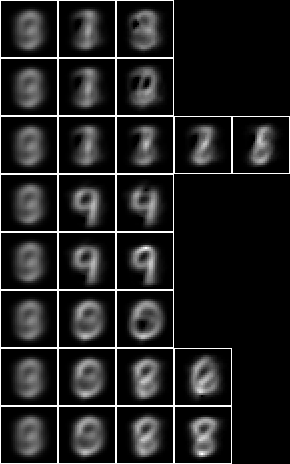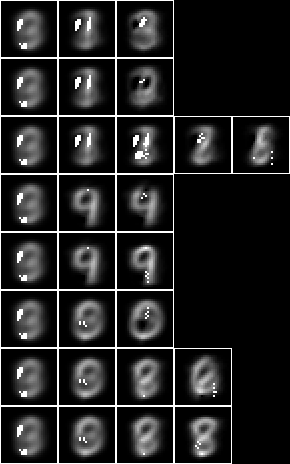Let us apply the 2-level 15-fud to the test sample to calculate the accuracy of prediction. First we will construct the dependent fud decomposition fud $F_2 = D_2^{‘\mathrm{F}}$,

let ff2 = dfnul uu2 df2' 2

card $fvars ff2 240 let uu2 = uu1 uunion (fsys ff2) card$ uvars uu2
1404


The underlying, $\mathrm{und}(F_2)$, is shown overlaid on the average, $\hat{A}\%V_{\mathrm{k}}$,

let hrbmav = hrbm 28 3 2 $hr hrhrred vvk bmwrite file$ bmborder 1 $bmmax hrbmav 0 0$ hrbm 28 3 2 $qqhr 2 uu vvk (fund ff2)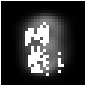We can see that the 2-level 15-fud covers more of the substrate than the 15-mono-variate-fud. Consider this model,$D’_2$, as a predictor of digit, let ww2 = fder ff2 let hr2 = hrfmul uu2 ff2 hr  The label entropy,$\mathrm{lent}(A * \mathrm{his}(F_2^{\mathrm{T}}),W_2,V_{\mathrm{l}})$, where$W_2 = \mathrm{der}(F_2)$, is hrlent uu2 hr2 ww2 vvl 1.2942177631075924  This model is effective for all of the test sample,$\mathrm{size}((A_{\mathrm{te}} * F_2^{\mathrm{T}}) * (A * F_2^{\mathrm{T}})^{\mathrm{F}}) \approx \mathrm{size}(A_{\mathrm{te}})$, let hrq2 = hrfmul uu2 ff2 hrq size$ hhaa (hrhh uu2 (hrq2 hrhrred ww2)) mul eff (hhaa (hrhh uu2 (hr2 hrhrred ww2)))
1000 % 1


It is correct for 54.1% of events, $\begin{eqnarray} &&|\{R : (S,\cdot) \in A_{\mathrm{te}} * \mathrm{his}(F_2^{\mathrm{T}})~\%~(W_2 \cup V_{\mathrm{l}}),~Q = \{S\}^{\mathrm{U}}, \\ &&\hspace{4em}R = A * \mathrm{his}(F_2^{\mathrm{T}})~\%~(W_2 \cup V_{\mathrm{l}}) * (Q\%W_2),~\mathrm{size}(\mathrm{max}(R) * (Q\%V_{\mathrm{l}})) > 0\}| \end{eqnarray}$

let amax = llaa . take 1 . reverse . map (\(a,b) -> (b,a)) . sort . map (\(a,b) -> (b,a)) . aall . norm . trim

length [rr | let hhq = hrhh uu2 (hrq2 hrhrred (ww2 union vvl)), let aa = hhaa (hrhh uu2 (hr2 hrhrred (ww2 union vvl))), (_,ss) <- hhll hhq, let qq = single ss 1, let rr = aa mul (qq red ww2) red vvl, size rr > 0, size (amax rr mul (qq red vvl)) > 0]
541


This is a little less accurate than the 1-tuple 15-fud (56.7%), but higher than just model 2 by itself (46.3%). The clusters of the 2-level conditional over induced model tend to be a bit more spread out than the 1-level induced model. Note that the pure 1-level conditional model only has a single pixel per fud, rather than clusters.

We can run the conditional entropy fud decomper in an engine. Given NIST_model2.json, the engine creates NIST_model36.json, see Model 36,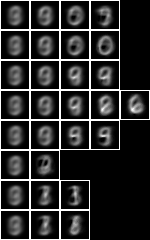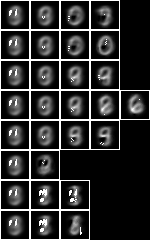We can then test the accuracy of model 36 in a compiled executable, see NIST test, with these results,

model: NIST_model36
selected train size: 7500
model cardinality: 206
nullable fud cardinality: 246
nullable fud derived cardinality: 15
nullable fud underlying cardinality: 87
ff label ent: 1.3402902066958342
test size: 1000
effective size: 1000 % 1
matches: 534


Note that the statistics differ slightly from those calculated above, because the engine uses the entire training dataset of 60,000 events.

### All pixels

Now let us repeat the analysis of Model 2, but for the 127-fud induced model of all pixels, see Model 35.

First let us test the accuracy of the induced model by itself in NIST test,

model: NIST_model35
selected train size: 7500
model cardinality: 3929
nullable fud cardinality: 5483
nullable fud derived cardinality: 1316
nullable fud underlying cardinality: 486
ff label ent: 0.7811149128042096
test size: 1000
effective size: 939 % 1
matches: 600


This model is correct for 60.0% of the test sample, which may be compared to Model 2 (46.3%). When compared to the non-modelled case it is more accurate than the 15-mono-variate-fud (56.7%), but less accurate than the 127-mono-variate-fud (70.2%).

Again, we can run the conditional entropy fud decomper to create a 2-level 127-fud, NIST_model37.json, see Model 37,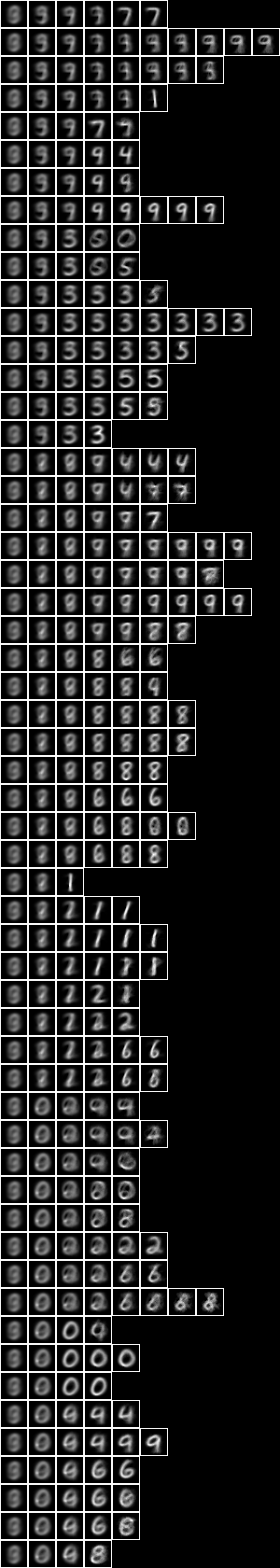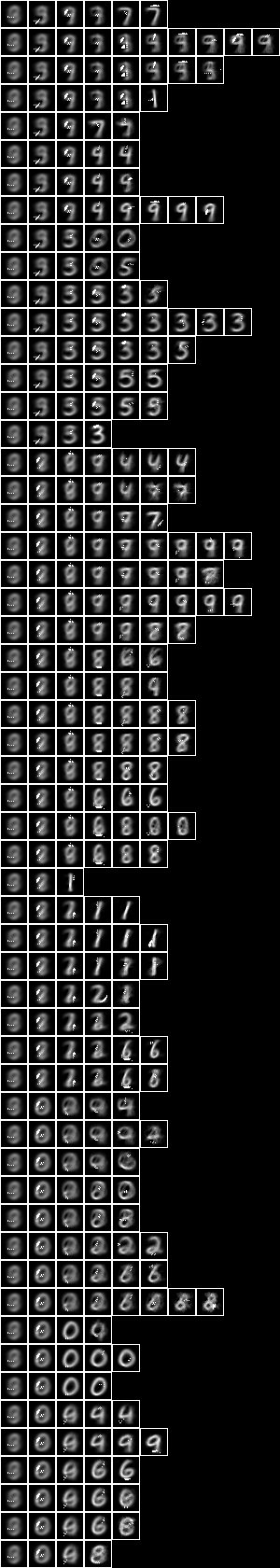Testing the accuracy of model 37,

model: NIST_model37
selected train size: 7500
model cardinality: 650
nullable fud cardinality: 1026
nullable fud derived cardinality: 127
nullable fud underlying cardinality: 255
ff label ent: 0.5951985228651324
test size: 1000
effective size: 999 % 1
matches: 814


So the accuracy of 81.4% is higher than the 127-bi-variate-fud (74.3%) and the 511-mono-variate-fud (75.0%). Here we have demonstrated that a semi-supervised induced model can be more predictive of label (81.4%) than either the unsupervised induced model, $D$, (60.0%) or the non-induced model (74.3%).

Again, the clusters of the conditional over induced model look a bit more spread out than the 1-level induced model.

Repeating the same, but to create a 2-tuple conditional over 127-fud, NIST_model39.json, see Model 39,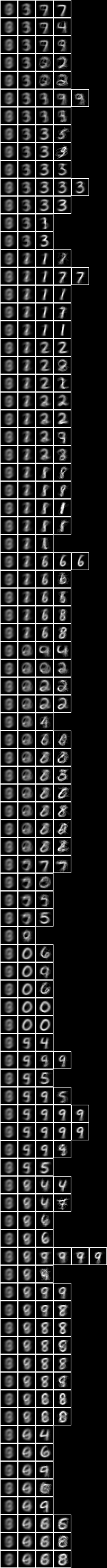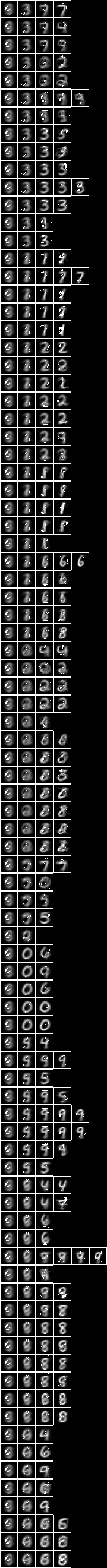Testing the accuracy of model 39,

model: NIST_model39
selected train size: 7500
model cardinality: 883
nullable fud cardinality: 1253
nullable fud derived cardinality: 127
nullable fud underlying cardinality: 322
ff label ent: 0.3877749488432780
test size: 1000
effective size: 985 % 1
matches: 824


The accuracy of the bi-variate has increased to 82.4%. It is not as great an increase as we saw between the 127-mono-variate-fud (70.2%) and the 127-bi-variate-fud (74.3%).

The clusters of the bi-variate conditional over induced model look still more spread out than the 1-level induced model](./dataset_NIST_induced_models#All_pixels).

The accuracy of the semi-supervised sub-model can be increased by obtaining larger samples, for example by random affine variation, and then inceasing the depth of the decomposition to 511-fud or 1023-fud.

### Averaged pixels

Now let us repeat the conditional entropy analysis for the 127-fud induced model of averaged pixels, see Model 34.

First let us test the accuracy of model 34 in NIST test averaged,

model: NIST_model34
selected train size: 7500
model cardinality: 4811
nullable fud cardinality: 6353
nullable fud derived cardinality: 1299
nullable fud underlying cardinality: 70
ff label ent: 0.6846668240290326
test size: 1000
effective size: 836 % 1
matches: 497


This model is correct for only 49.7% of the test sample, which is much less than Model 35 (60.0%).

Again, we can run the conditional entropy fud decomper to create a 2-level 127-fud, NIST_model38.json, see Model 38,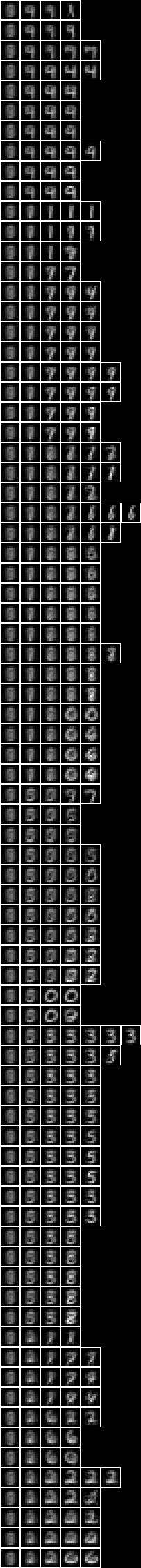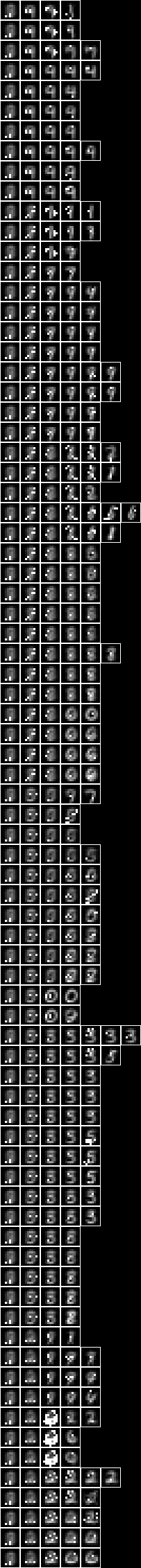Testing the accuracy of model 38,

model: NIST_model38
selected train size: 7500
model cardinality: 419
nullable fud cardinality: 794
nullable fud derived cardinality: 127
nullable fud underlying cardinality: 46
ff label ent: 0.6267873188842659
test size: 1000
effective size: 990 % 1
matches: 701


So the accuracy of the semi-supervised induced model, 70.1%, is higher than that of the unsupervised induced model, $D$, (49.7%), but not as high as the non-averaged all pixels model (81.4%).

### Array of square regions of 15x15 pixels

Now let us repeat the conditional entropy analysis of all pixels, but for a model consisting of an array of 7x7 127-fud induced models of square regions of 15x15 pixels, see Model 21.

When we run the regional conditional entropy fud decomper to create a conditional model, NIST_model43.json, see Model 43,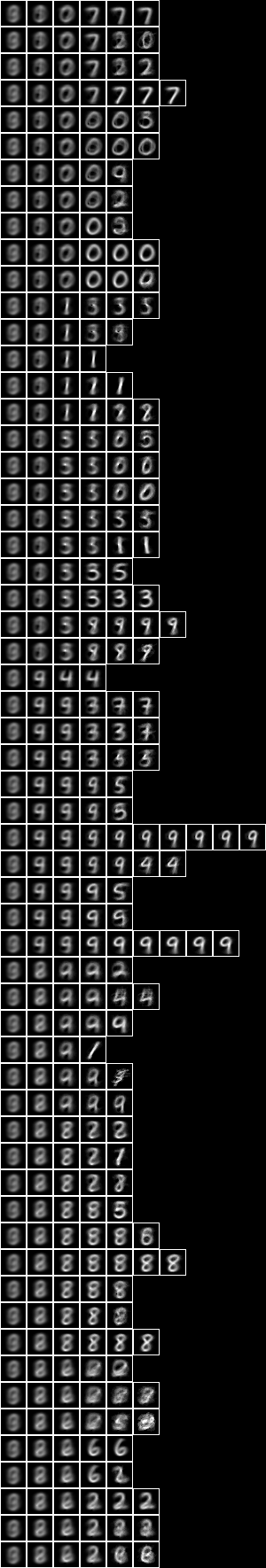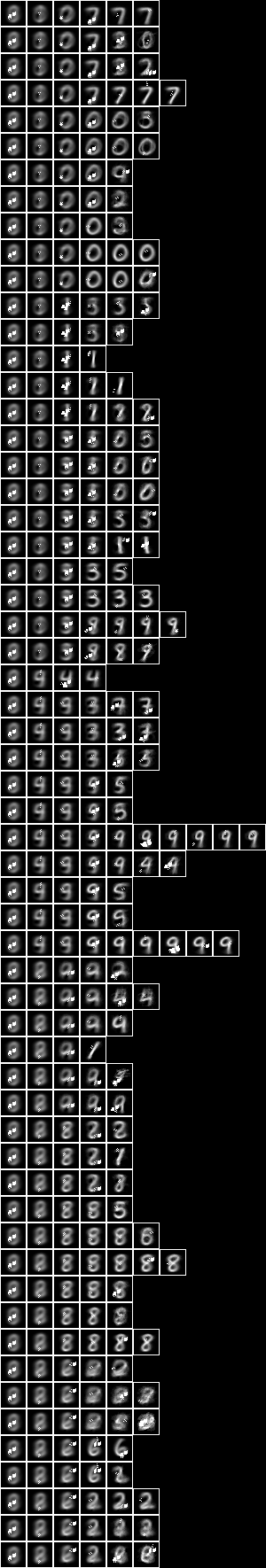Testing the accuracy of model 43,

model: NIST_model43
selected train size: 7500
model cardinality: 2034
nullable fud cardinality: 2410
nullable fud derived cardinality: 127
nullable fud underlying cardinality: 361
ff label ent: 0.5642948913994976
test size: 1000
effective size: 997 % 1
matches: 808


The accuracy of 80.8% is similar to the all pixels (81.4%) above.

### Two level over 10x10 regions

Now let us repeat the conditional entropy analysis of all pixels, but for the 2-level model over square regions of 10x10 pixels, see Model 24.

When we run the conditional entropy fud decomper to create a conditional model, NIST_model40.json, see Model 40,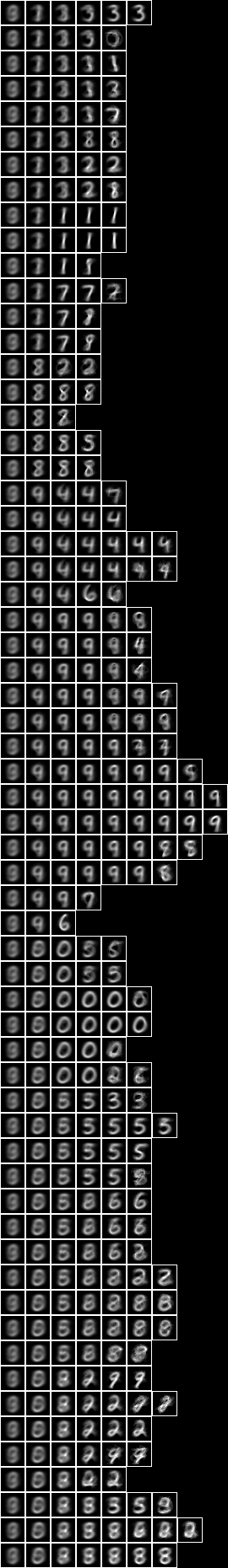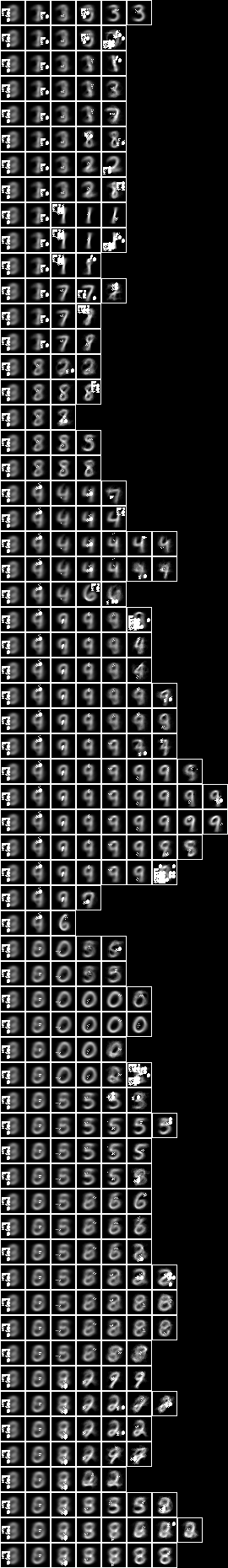Testing the accuracy of model 40,

model: NIST_model40
selected train size: 7500
model cardinality: 2296
nullable fud cardinality: 2671
nullable fud derived cardinality: 127
nullable fud underlying cardinality: 589
ff label ent: 0.5850540595121823
test size: 1000
effective size: 1000 % 1
matches: 794


The accuracy of 79.4% is similar to the all pixels (81.4%) above.

### Two level over 15x15 regions

Now let us repeat the conditional entropy analysis of all pixels, but for the 2-level model over square regions of 15x15 pixels, see Model 25.

When we run the conditional entropy fud decomper to create a conditional model, NIST_model41.json, see Model 41,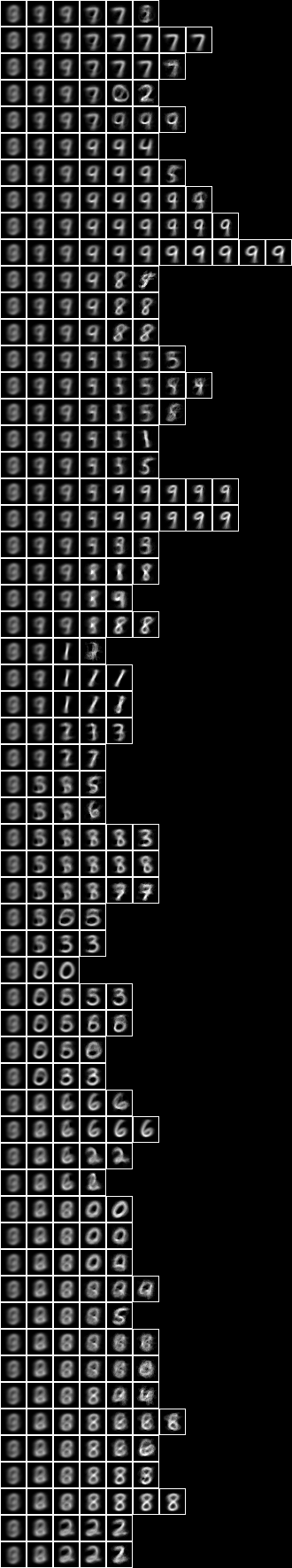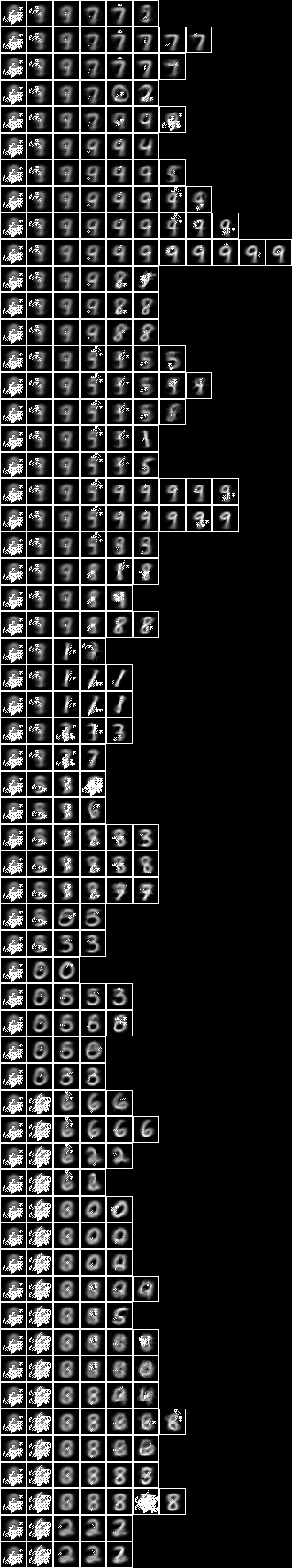Testing the accuracy of model 41,

model: NIST_model41
selected train size: 7500
model cardinality: 1522
nullable fud cardinality: 1897
nullable fud derived cardinality: 127
nullable fud underlying cardinality: 452
ff label ent: 0.5974764620331507
test size: 1000
effective size: 994 % 1
matches: 791


The accuracy of 79.1% is similar to the all pixels (81.4%) and the two level over 10x10 regions (79.4%) above.

### Two level over centred square regions of 11x11 pixels

Now let us repeat the conditional entropy analysis of all pixels, but for the 2-level model over centred square regions of 11x11 pixels, see Model 26.

When we run the conditional entropy fud decomper to create a conditional model, NIST_model42.json, see Model 42,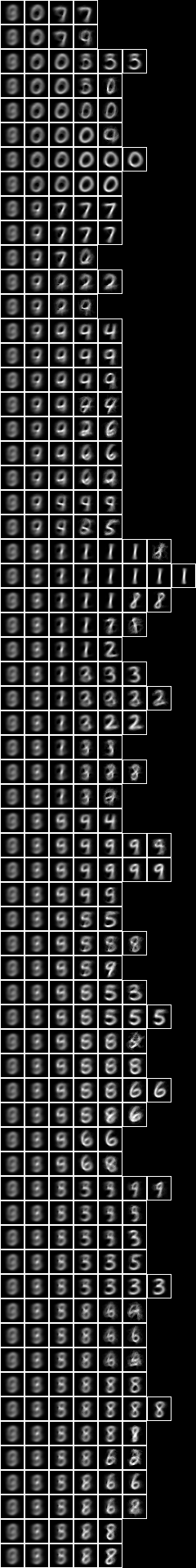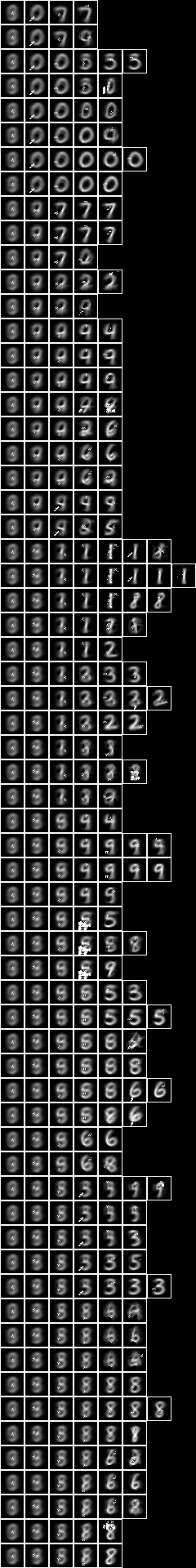Testing the accuracy of model 42,

model: NIST_model42
selected train size: 7500
model cardinality: 835
nullable fud cardinality: 1211
nullable fud derived cardinality: 127
nullable fud underlying cardinality: 311
ff label ent: 0.6526213410903168
test size: 1000
effective size: 1000 % 1
matches: 786


The accuracy of 78.6% is similar to the all pixels (81.4%), the two level over 10x10 regions (79.4%), and the two level over 15x15 regions (79.1%) above.

In general, although the 2-level induced models that are based on underlying regional levels have higher alignments than the 1-level induced model, these extra alignments are not particularly related to digit, and so the higher level features are not very prominent in the resulting semi-supervised sub-models.

top